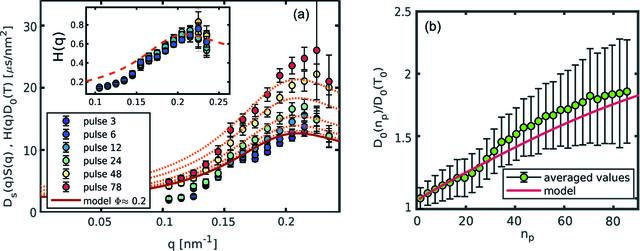disable zoom     view article Figure 5 Dynamical quantities as a function of the number of pulses for sample A exposed to 10.5 mJ mm−2. (a) Contribution of the HIs to the diffusion constant for various np. The lines are the equilibrium Hm(q) multiplied by the expected diffusion constant. In the inset the data points are rescaled by the expected diffusion constant and plotted together with the equilibrium Hm(q). (b) Relative change in the diffusion constant; the values are obtained from the average over q of, and the error bars from the propagation of the uncertainty of the fitted relaxation rates, expressing a systematic error originating from a reduced time resolution at larger np. The red line is the change in D0(T) as expected from the time-resolved model by Lehmkühler et al. (2020).

IUCrJ
Volume 8| Part 5| September 2021| Pages 775-783
ISSN: 2052-2525